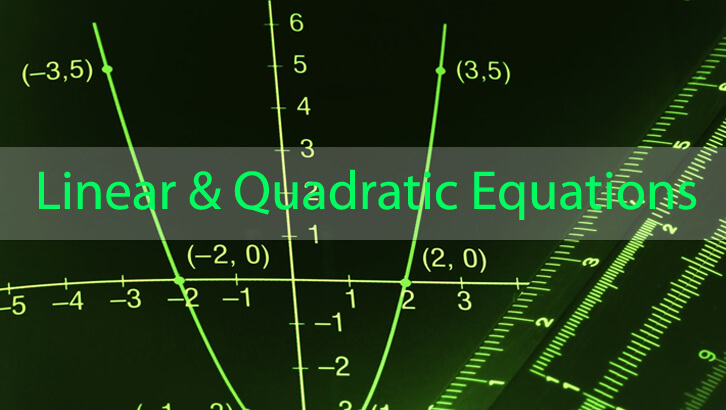# CAT Linear Equations

Question
(|x| -3) (|y| + 4) = 12. How many pairs of integers (x, y) satisfy this equation?
1. 4
2. 10
3. 6
4. 8